# Summation of Divergent Series and Integration of Divergent Integrals

The following article is from The Great Soviet Encyclopedia (1979). It might be outdated or ideologically biased.

## Summation of Divergent Series and Integration of Divergent Integrals

The attribution of some generalized sum to an infinite series that lacks an ordinary sum is known as the summation of a divergent series. The integration of a divergent integral, which lacks a definite value, involves the attribution of some value to the integral. Divergent series may be obtained when conditionally convergent series are multiplied, when functions are expanded in Fourier series, when series of functions are differentiated or integrated, and so on. Divergent series and integrals are often encountered in various problems in modern physics—for example, in the theory of electromagnetic fields.

In many cases, a sum can be assigned to a divergent series or a value can be associated with a divergent integral. In other words, a sum or value, in some generalized sense, can be found that has some of the basic properties of the ordinary sum of a convergent series or the ordinary value of a convergent integral. Usually, if the sum of the series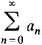is S and the sum of the series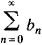is T, it is required that the sum of the series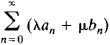be λS μT and that the sum of the series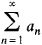be Sa0. Moreover, regular methods of summation are most often considered—that is, methods that yield for every convergent series its ordinary sum.

In most summation methods, a divergent series is regarded as the limit, in some sense, of a convergent series. More precisely, every term of the series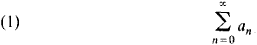is multiplied by some factor λn(t) such that a convergent series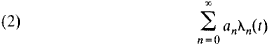with sum δ(t) is then obtained. The factors λn(t) are chosen so that, for every fixed n, the limit of λn(t) is 1 for some continuous or discrete variation of the parameter t. The terms of series (2) will then tend to the corresponding terms of series (1). If δ(t) has a limit, this limit is called the generalized sum, of the given series, corresponding to the given choice of the factor or to the given method of summation. Suppose, for example, λn(t) = 1 for nt and λn(t) = 0 for n > t. If t → ∞ the ordinary concept of the sum of a series is obtained. The Abel summation method is obtained when λn(t) = tn, for t < 1, and t → 1.

Some summation methods are concerned not with the result of the multiplication of the terms of a series by λn(t) but with the corresponding changes in the partial sums of the series. For example, in the method of arithmetic means, which is also known as the Cesàro method of order 1,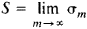where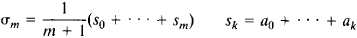This method corresponds to the choice λm = (mn + 1)/(m + 1) for n ≤ m and λn(m) = 0 for n > m. Suppose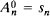and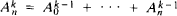; furthermore, let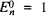and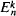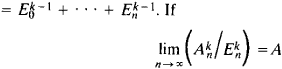exists, the series is said to be summable to A by the Cesàro method of order k. Cesàro methods of fractional order are also considered. The power of the Cesàro method increases with increasing k—that is, the set of series summable by the method expands as k increases.

Every series that is summable by a Cesàro method of some order is also summable by the Abel method; moreover, the sum is the same. For example, the series 1 – 1 + 1– ... + (–1)n–1+ ... is summable to ½ by the Abel method, since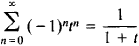and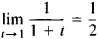The Cesàro method yields the same value, since s2n = 1, s2n+1 = 0, σ2n = (n + 1)/(2n + 1), σ2n+1 = ½, and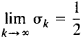The Cesàro and Abel methods are used in the theory of trigonometric series to determine functions in terms of their Fourier expansions, since the Fourier series of any continuous function is summable to the function by the Cesàro method of order 1 and, consequently, by the Abel method.

In 1901, G. F. Voronoi proposed a summation method that includes all the Cesàro methods as special cases. Suppose pn ≥ 0, p0 = 0, and Pn = p0 + p1 + ... + pn. The limit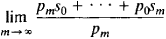is called the generalized Voronoi sum of the series. The Voronoi method is regular if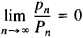In 1911, the German mathematician O. Toeplitz found necessary and sufficient conditions that must be satisfied by the triangular matrix ║amn║, where amn = 0 when n > m, in order for the summation method defined by the formula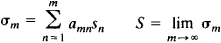to be regular. The Polish mathematician H. Steinhaus generalized these conditions to the case of square matrices.

An important role is played in the theory of analytic functions by the Borel summation method, which permits a function defined by a power series to be analytically continued outside the circle of convergence. S. N. Bernshtein and the German mathematician W. Rogosinski proposed an important summation method for trigonometric series. Bernshtein used the method to obtain convergent interpolation processes.

The theory of the integration of divergent integrals is similar to the theory of the summation of divergent series. For example, if the integral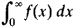diverges and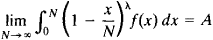exists, the first integral is said to be summable to A by the Cesàro method of order λ.